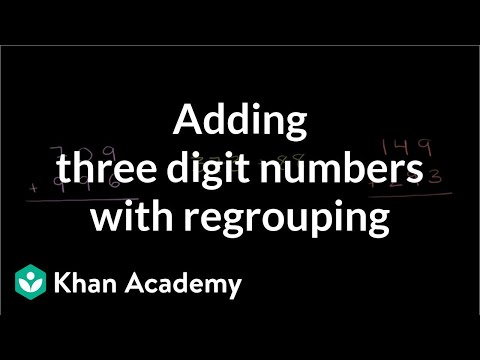Video

# Adding and subtracting on the number line (Full video)

Description: Sal adds and subtracts numbers like 585 and 368 using a number line. Numbers used in these problems are less than 1000. Let's see, in all of them we're starting at 361, so now let's add 544. This one over here, instead of add 500, you add 50, which doesn't make sense because we're clearly adding 5 hundreds, 4 tens, or 40, and then 4 ones, let's do some more examples. Let's see, we're starting at 718, and then we're adding 200, and then we're adding 40 to get to 958.

### Other videos you might be interested in### Adding 3-digit numbers with regrouping (Full video)